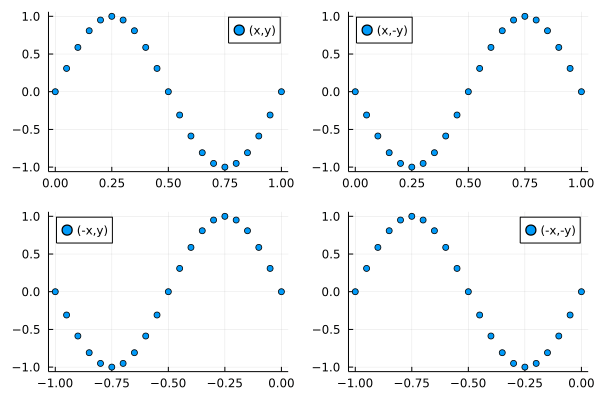To compile multiple plots into a single figure, we need to adjust the layout.

There are many ways to do this, and arguably the simplest one is to create the plots sequentially, as follows:

using Plots
p1 = scatter(x, y, label="(x,y)")
p2 = scatter(x, -y, label="(x,-y)", legend=:topleft)
p3 = scatter(-x, y, label="(-x,y)", legend=:topleft)
p4 = scatter(-x, -y, label="(-x,-y)")    

and then combining them into a single plot with

plot(p1,p2,p3,p4, layout = (2,2) )

This is the result: